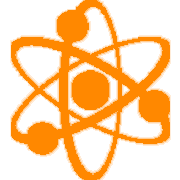SCI-HS.PS3.01High School (SCI) Physical Science Standards
[PS3] Energy

SCI-HS.PS3.01 Create a mathematical model to calculate the change in the energy of one component in a system when the change in energy of the other component(s) and energy flows in and out of the system are known.

Clarification Statement:
Physical Science: Emphasis is on basic algebraic expressions or computations; to systems of two or three components; and to thermal energy, kinetic energy, and potential energy.
Chemistry: Emphasis is on explaining the meaning of mathematical expressions used in the model. Focus is on basic algebraic expression or computations, systems of two or three components, and thermal energy.
Physics: Emphasis is on explaining the meaning of mathematical expressions used in the model. Focus is on basic algebraic expression or computations; systems of two or three components; and thermal energy, kinetic energy, and/or the energies in gravitational, magnetic, or electric fields

Disciplinary Core Ideas
PS3.A: Definitions of Energy A system’s total energy is conserved within its system and surroundings.
PS3.B: Conservation of Energy and Energy Transfer Energy cannot be created or destroyed, but it can be transferred. Mathematical expressions, including potential and kinetic energy allow the concept of conservation of energy to be used to describe a system. The availability of energy limits what can occur in any system.

• I can

• I can

• I can

• I can

Proficiency Scale

1 Beginning
... with help, demonstrate a partial understanding of some of the simpler details and processes (Score 2.0 content) and some of the more complex ideas and processes (Score 3.0 content).
• descriptors
2 Developing
... demonstrate no major errors or omissions regarding the simpler details and processes but exhibits major errors or omissions regarding the more complex ideas and processes (Score 3.0 content).
• descriptors
3 Proficient
“The Standard.”
... demonstrate no major errors or omissions regarding any of the information and processes that were end of instruction expectations.
• descriptors